# NCERT Exemplar Class 11 Physics Solutions for Chapter 9 - Mechanical Properties Of Solids

NCERT exemplar class 11 physics Chapter 9 Mechanical Properties of Solids make you ready for the CBSE class 11 board exam and all important graduate entrance examinations. This chapter is very important from the exam point for it has a good allotment of marks.
NCERT exemplar class 11 physics Chapter 9 pdf provide exemplar question from NCERT exemplar book, previous year question papers, sample papers, extra questions prepared by subject experts at BYJU’S. A solid is the strongest state of matter when compared to gases and liquids. Understanding the mechanical properties of solids is important in understanding the characteristics of the material of the solid. To change the shape and size of an object, an external force needed to be applied.

## Important topics of class 11 physics chapter 9 Mechanical Properties of Solids

1. Elastic behaviour of solids
2. Stress and strain
3. Hooke’s law
4. Stress-strain curve
5. Elastic Moduli
6. Applications of elastic behaviour of materials.

## Download NCERT exemplar class 11 physics Chapter 9 Mechanical Properties of Solids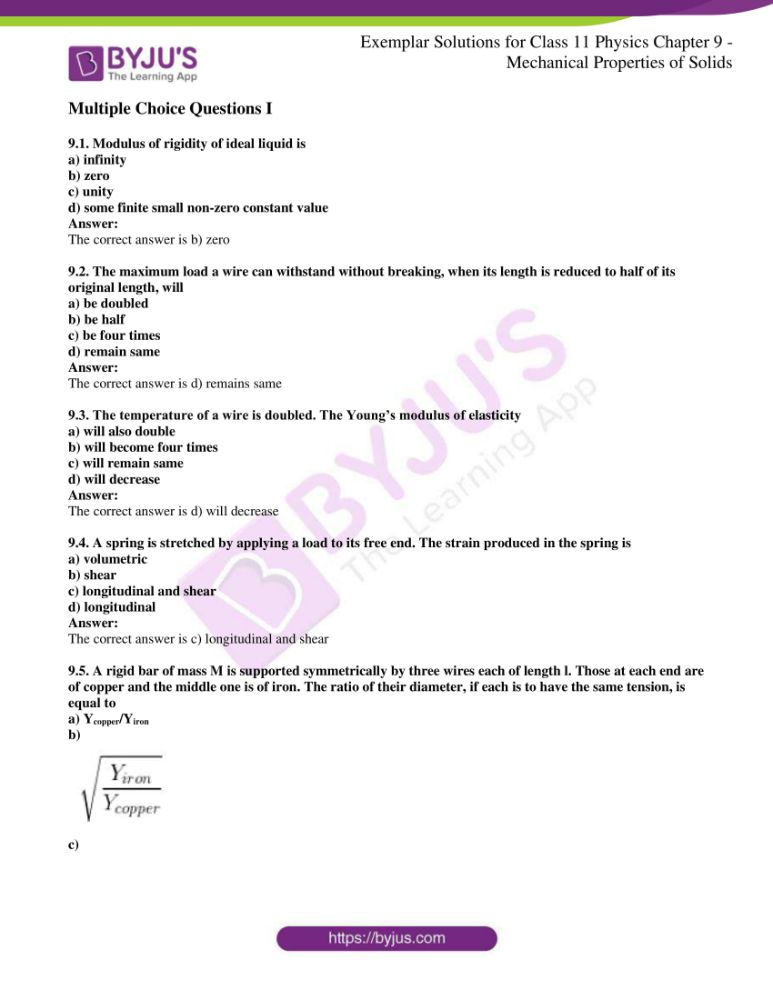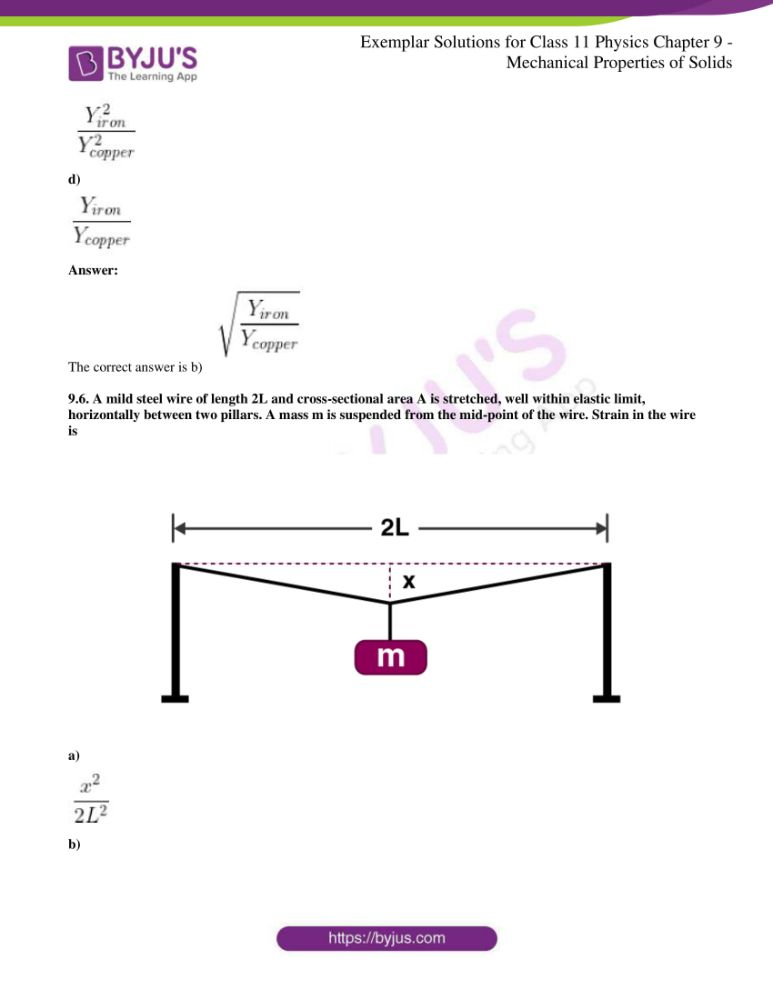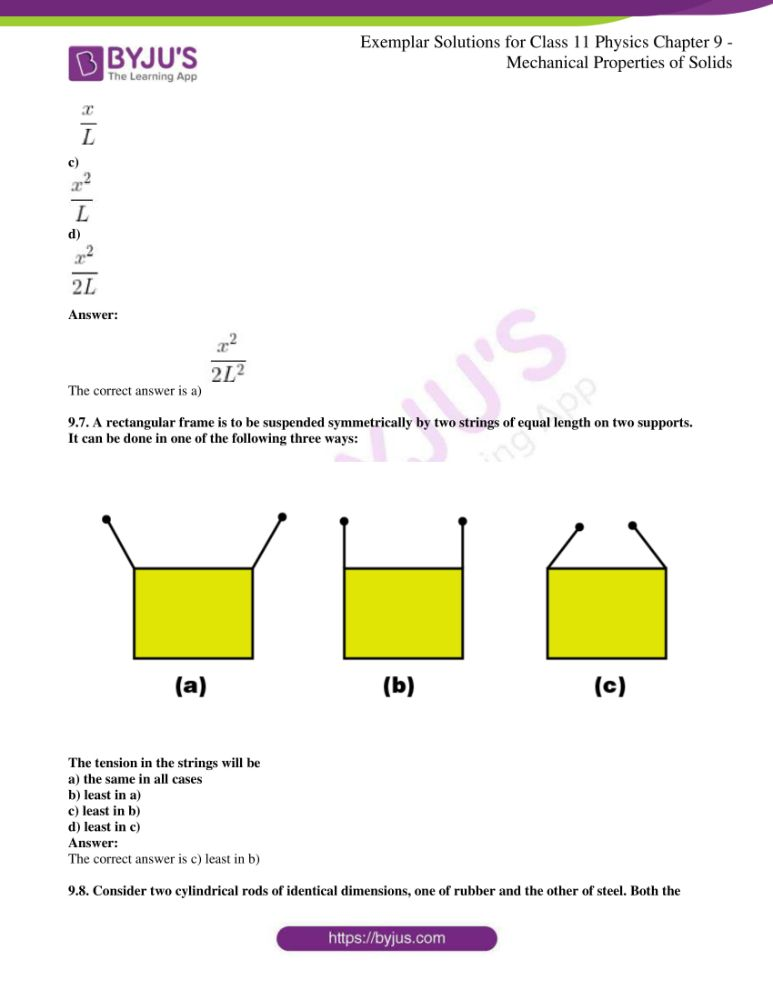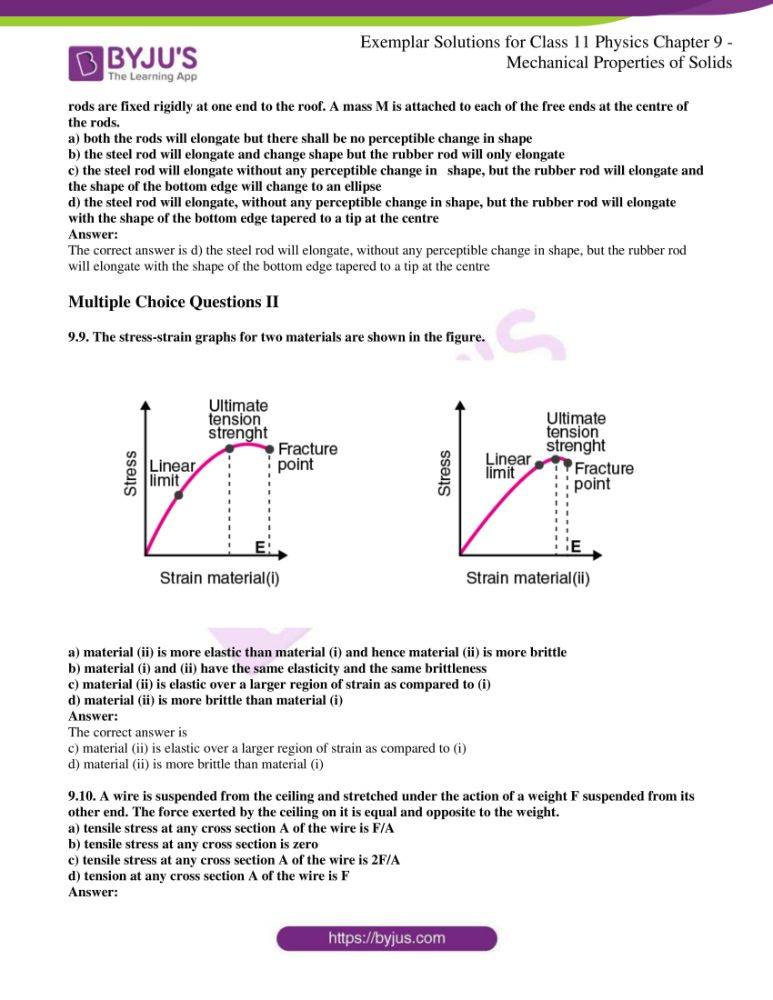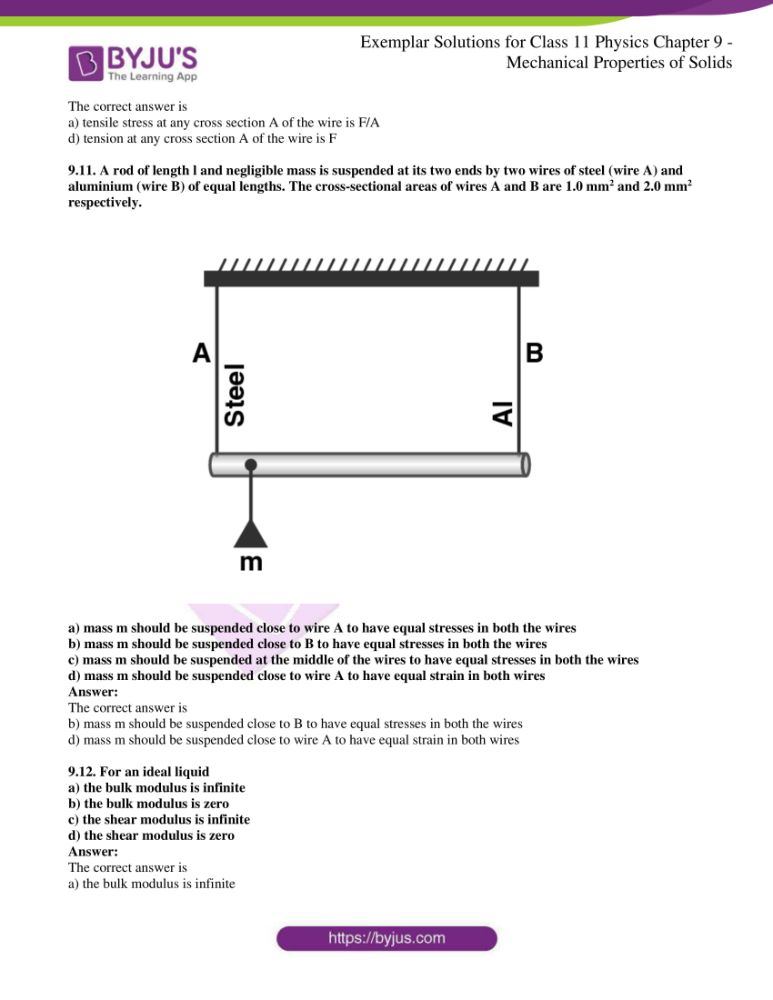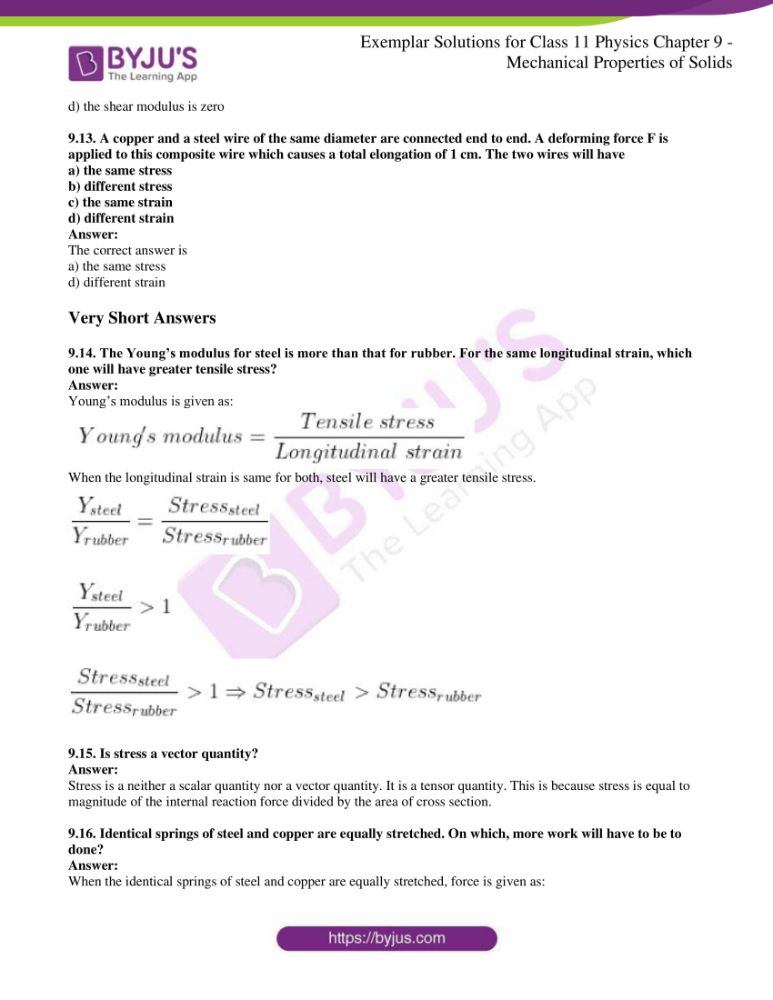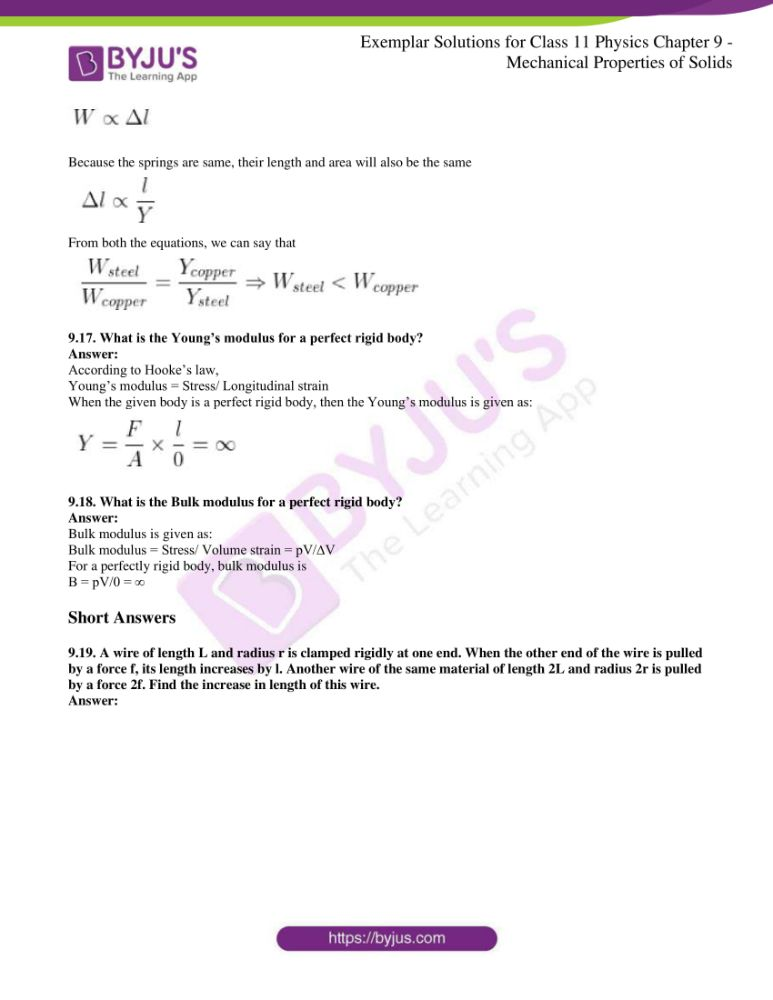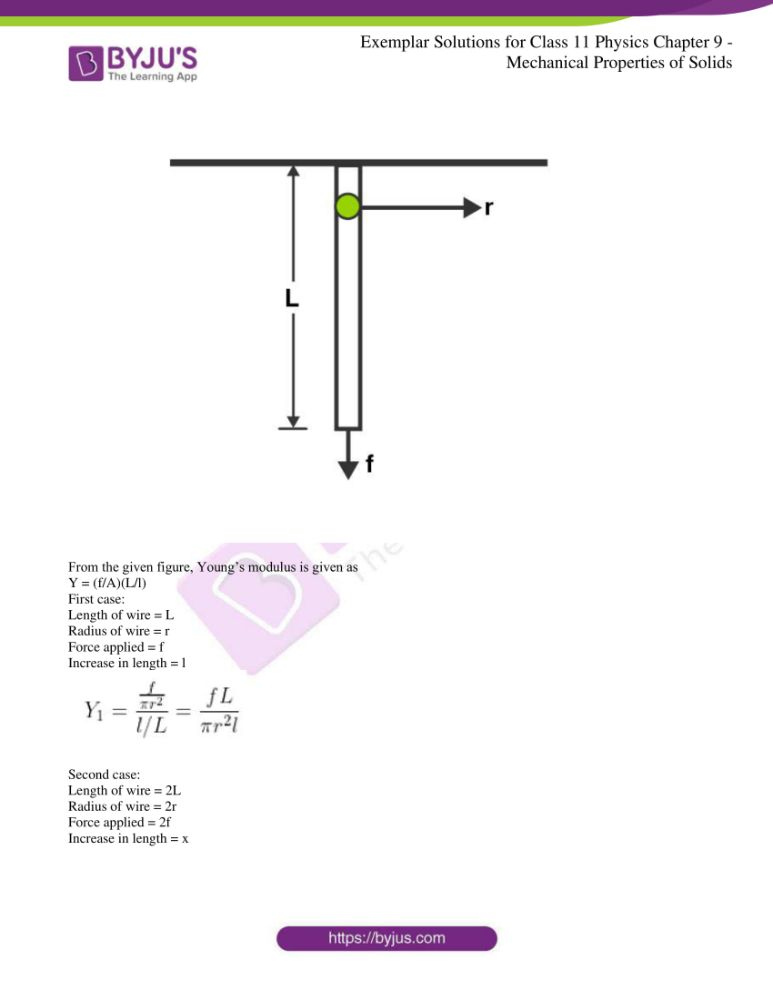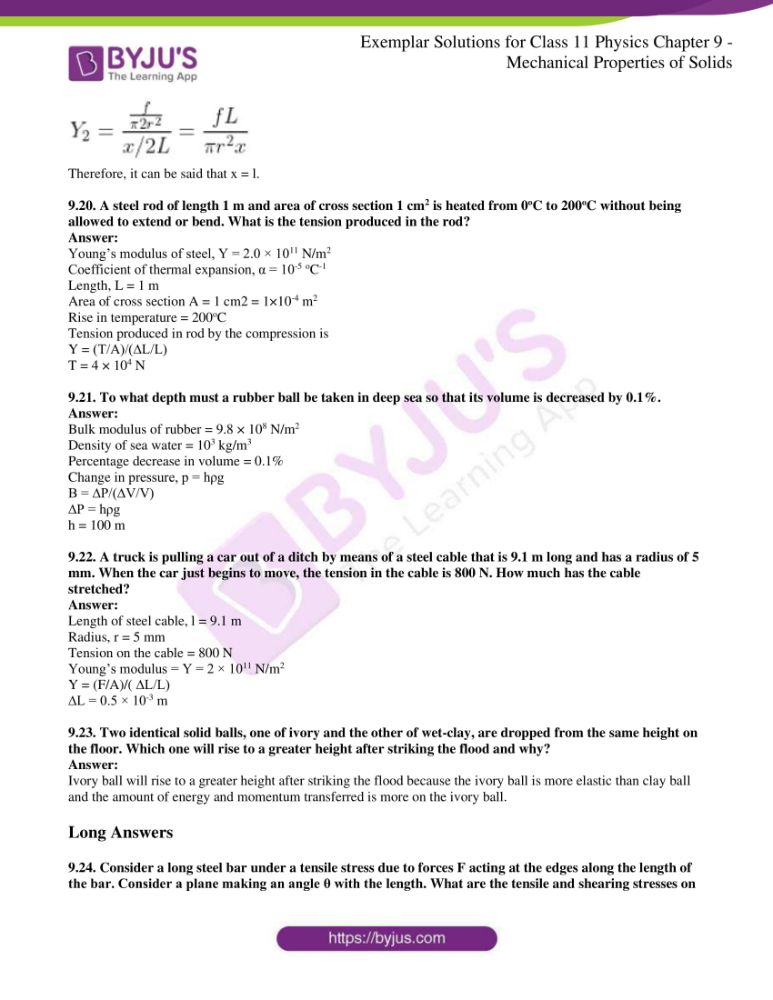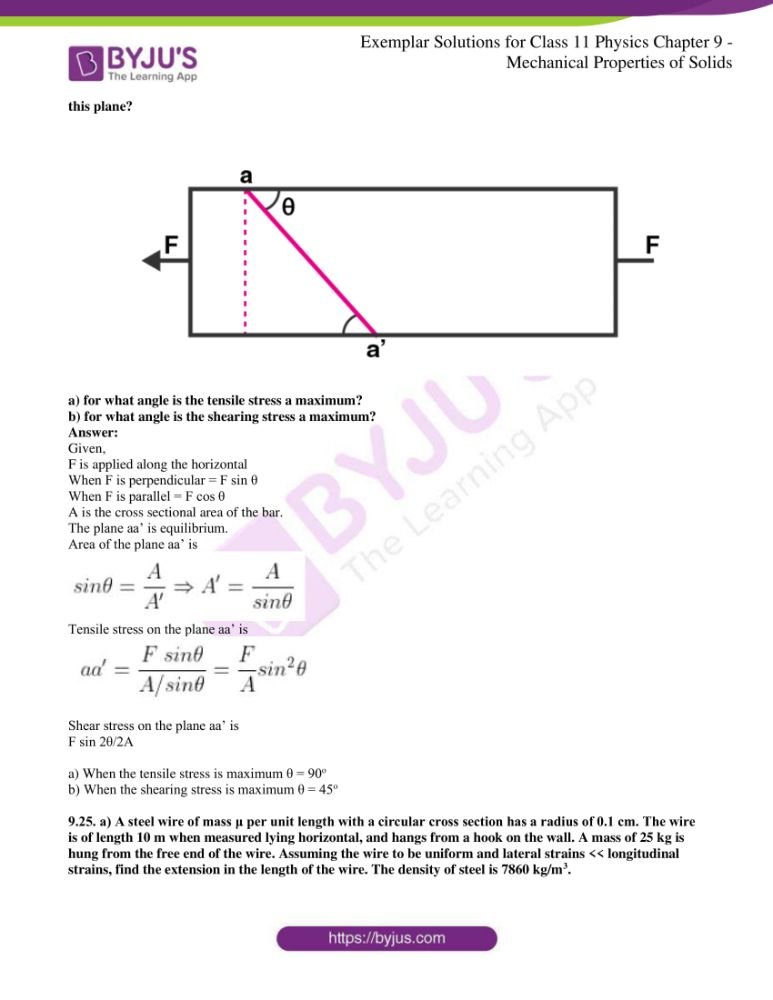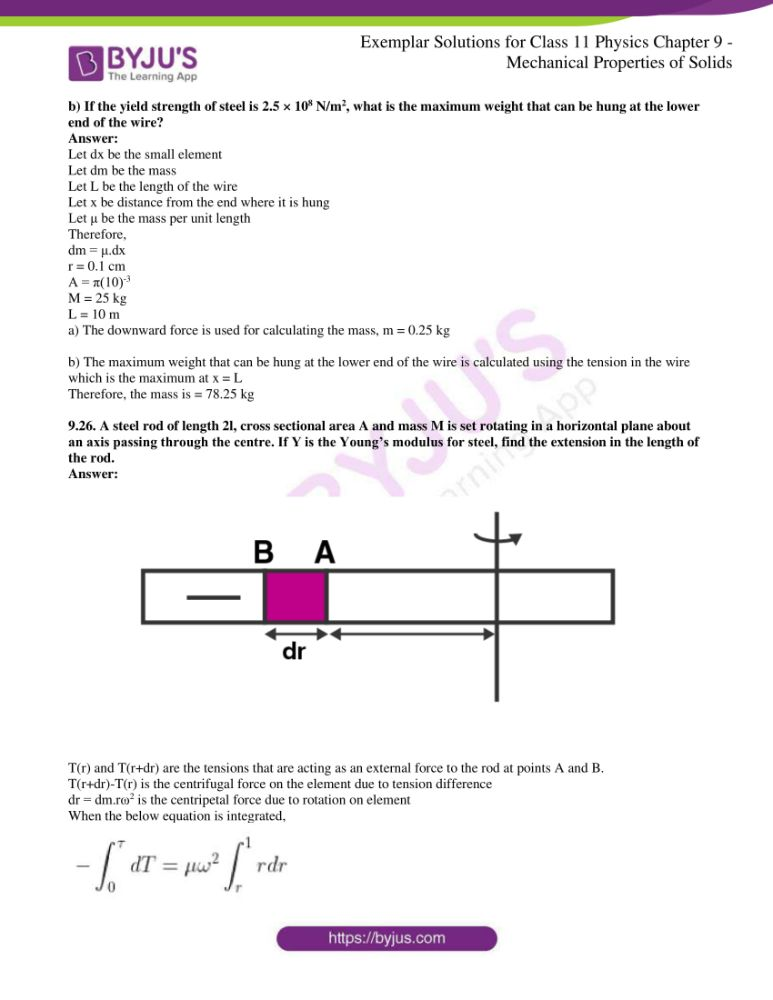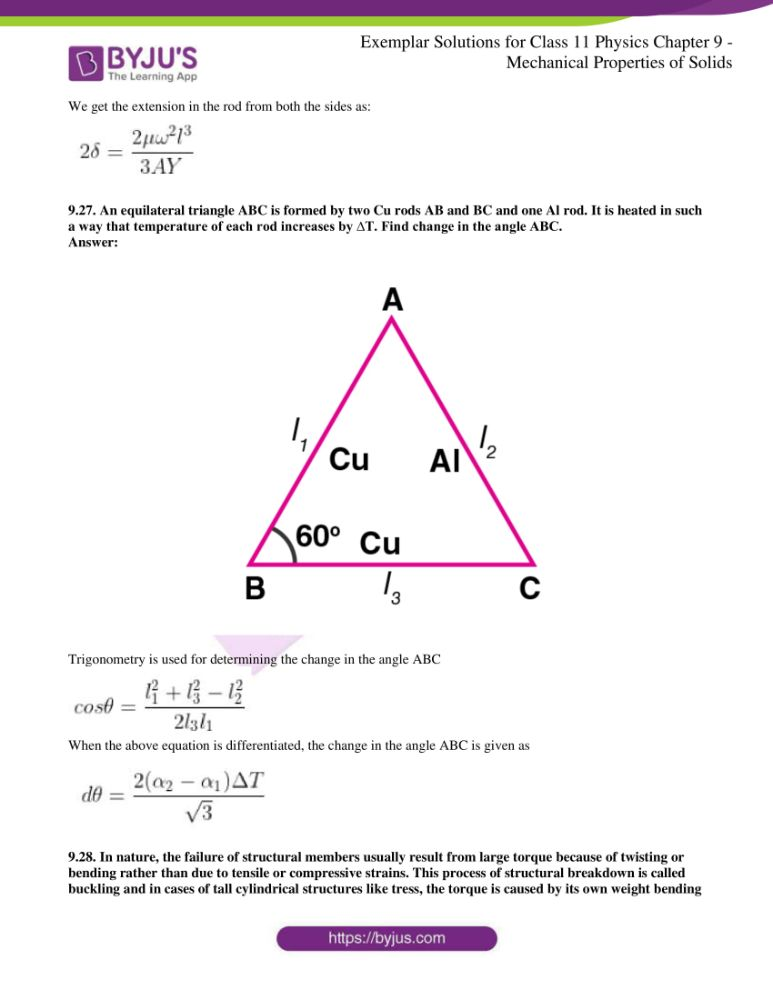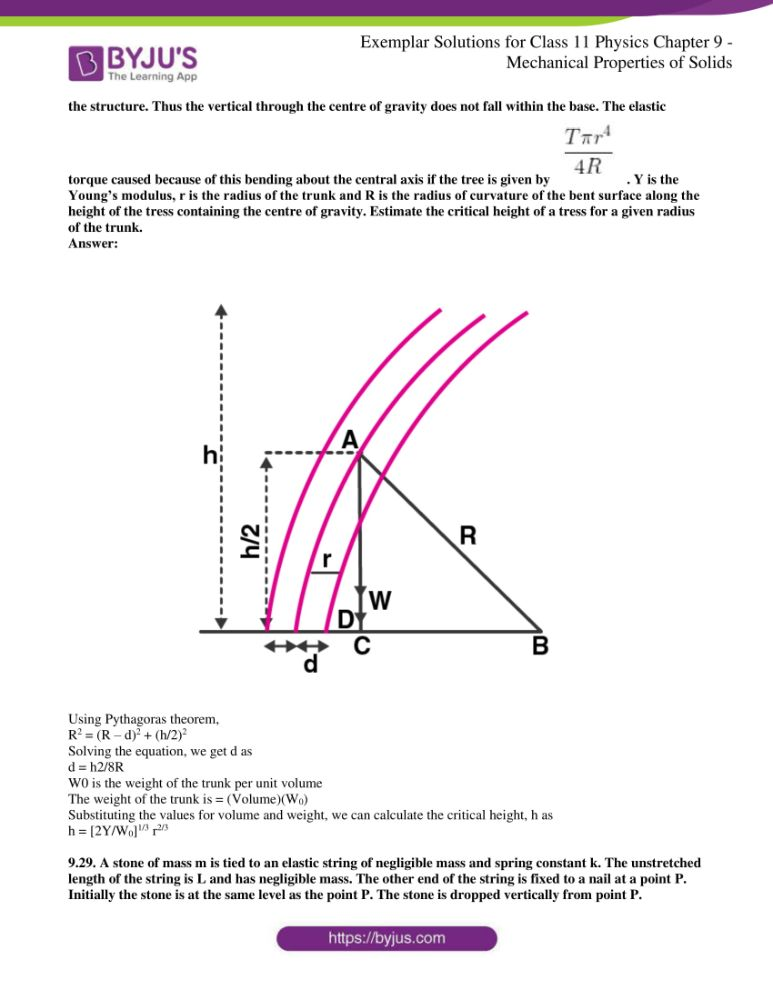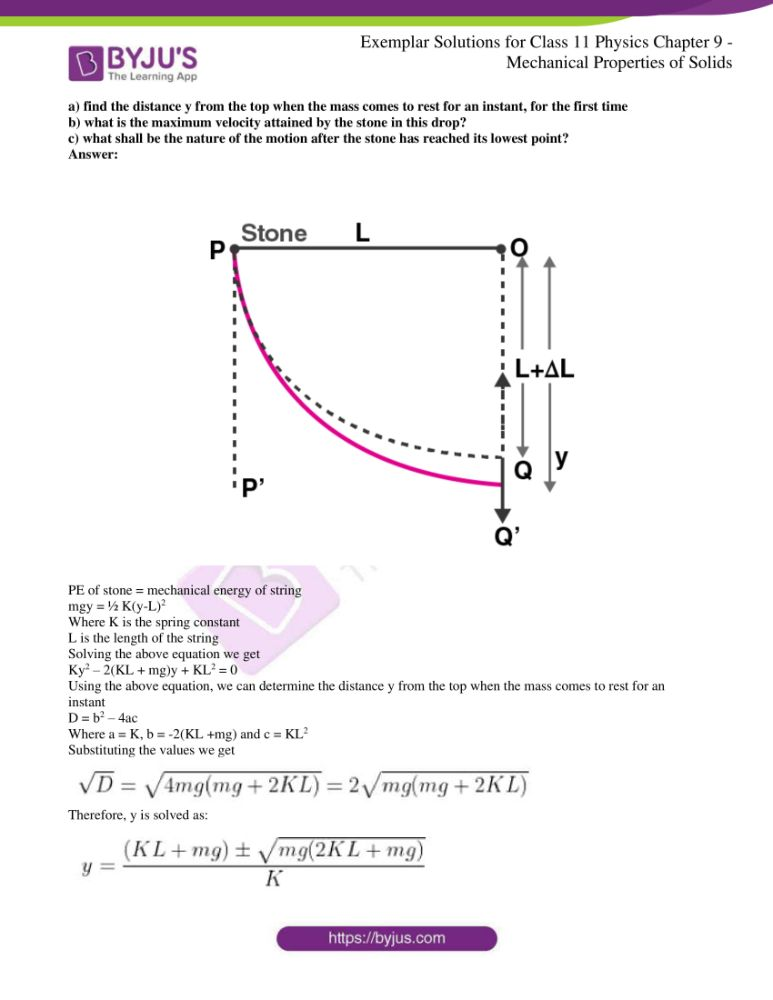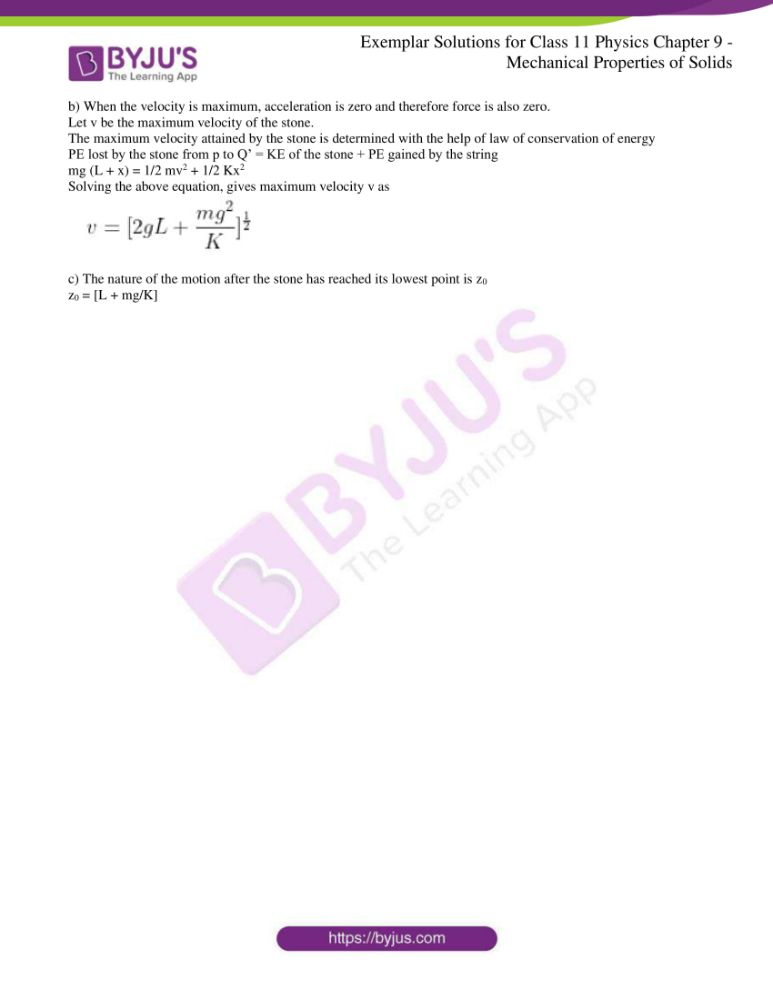### Multiple Choice Questions I

9.1. Modulus of rigidity of ideal liquid is

a) infinity

b) zero

c) unity

d) some finite small non-zero constant value

The correct answer is b) zero

9.2. The maximum load a wire can withstand without breaking when its length is reduced to half of its original length, will

a) be doubled

b) be half

c) be four times

d) remain same

The correct answer is d) remains same

9.3. The temperature of a wire is doubled. The Young’s modulus of elasticity

a) will also double

b) will become four times

c) will remain same

d) will decrease

The correct answer is d) will decrease

9.4. A spring is stretched by applying a load to its free end. The strain produced in the spring is

a) volumetric

b) shear

c) longitudinal and shear

d) longitudinal

The correct answer is c) longitudinal and shear

9.5. A rigid bar of mass M is supported symmetrically by three wires each of length l. Those at each end are of copper and the middle one is of iron. The ratio of their diameter, if each is to have the same tension, is equal to

a) Ycopper/Yiron

b)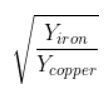c)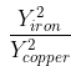d)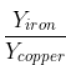9.6. A mild steel wire of length 2L and cross-sectional area A is stretched, well within elastic limit, horizontally between two pillars. A mass m is suspended from the mid-point of the wire. Strain in the wire is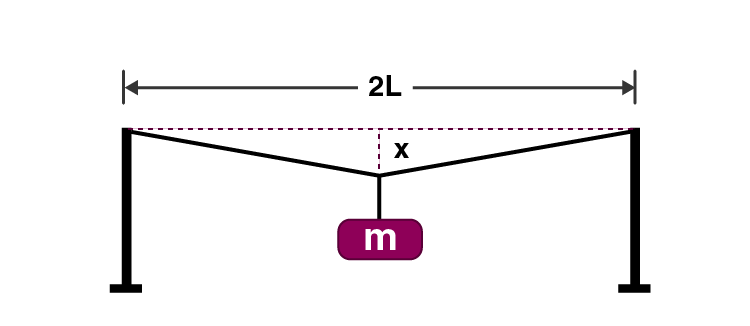a)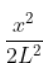b)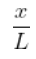c)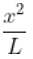d)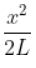9.7. A rectangular frame is to be suspended symmetrically by two strings of equal length on two supports. It can be done in one of the following three ways: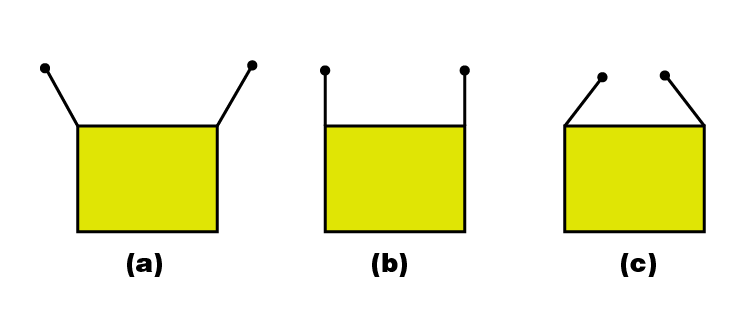The tension in the strings will be

a) the same in all cases

b) least in a)

c) least in b)

d) least in c)

The correct answer is c) least in b)

9.8. Consider two cylindrical rods of identical dimensions, one of rubber and the other of steel. Both the rods are fixed rigidly at one end to the roof. A mass M is attached to each of the free ends at the centre of the rods.

a) both the rods will elongate but there shall be no perceptible change in shape

b) the steel rod will elongate and change shape but the rubber rod will only elongate

c) the steel rod will elongate without any perceptible change in shape, but the rubber rod will elongate and the shape of the bottom edge will change to an ellipse

d) the steel rod will elongate, without any perceptible change in shape, but the rubber rod will elongate with the shape of the bottom edge tapered to a tip at the centre

The correct answer is d) the steel rod will elongate, without any perceptible change in shape, but the rubber rod will elongate with the shape of the bottom edge tapered to a tip at the centre

### Multiple Choice Questions II

9.9. The stress-strain graphs for the two materials are shown in the figure.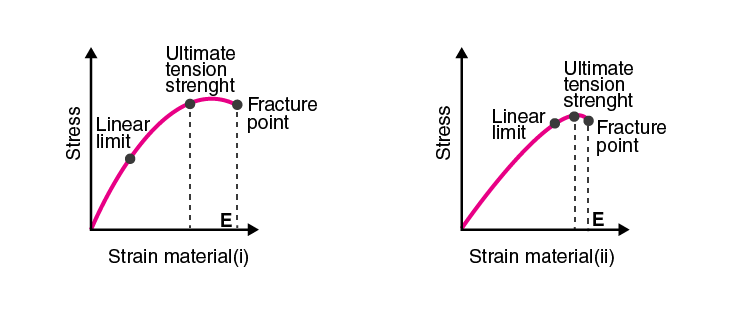a) material (ii) is more elastic than material (i) and hence material (ii) is more brittle

b) material (i) and (ii) have the same elasticity and the same brittleness

c) material (ii) is elastic over a larger region of strain as compared to (i)

d) material (ii) is more brittle than material (i)

c) material (ii) is elastic over a larger region of strain as compared to (i)

d) material (ii) is more brittle than material (i)

9.10. A wire is suspended from the ceiling and stretched under the action of a weight F suspended from its other end. The force exerted by the ceiling on it is equal and opposite to the weight.

a) tensile stress at any cross-section A of the wire is F/A

b) tensile stress at any cross-section is zero

c) tensile stress at any cross-section A of the wire is 2F/A

d) tension at any cross-section A of the wire is F

a) tensile stress at any cross-section A of the wire is F/A

d) tension at any cross-section A of the wire is F

9.11. A rod of length l and negligible mass is suspended at its two ends by two wires of steel (wire A) and aluminium (wire B) of equal lengths. The cross-sectional areas of wires A and B are 1.0 mm2 and 2.0 mm2 respectively.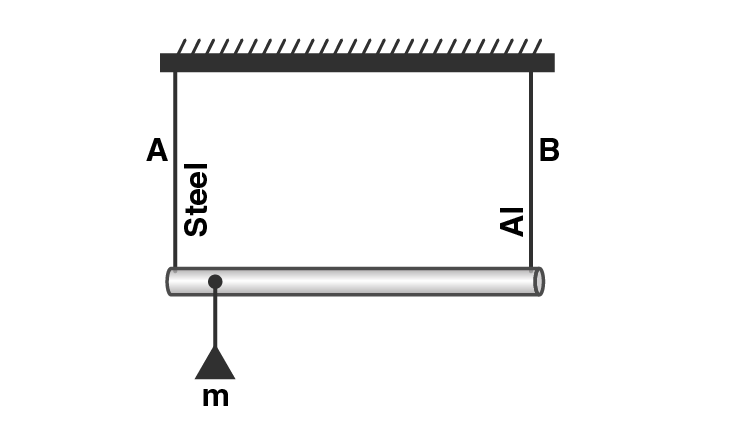a) mass m should be suspended close to wire A to have equal stresses in both the wires

b) mass m should be suspended close to B to have equal stresses in both the wires

c) mass m should be suspended at the middle of the wires to have equal stresses in both the wires

d) mass m should be suspended close to wire A to have equal strain in both wires

b) mass m should be suspended close to B to have equal stresses in both the wires

d) mass m should be suspended close to wire A to have equal strain in both wires

9.12. For an ideal liquid

a) the bulk modulus is infinite

b) the bulk modulus is zero

c) the shear modulus is infinite

d) the shear modulus is zero

a) the bulk modulus is infinite

d) the shear modulus is zero

9.13. A copper and a steel wire of the same diameter are connected end to end. A deforming force F is applied to this composite wire which causes a total elongation of 1 cm. The two wires will have

a) the same stress

b) different stress

c) the same strain

d) different strain

a) the same stress

d) different strain

9.14. The Young’s modulus for steel is more than that for rubber. For the same longitudinal strain, which one will have greater tensile stress?

Young’s modulus is given as: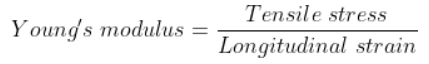When the longitudinal strain is the same for both, steel will have a greater tensile stress.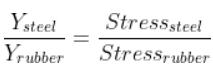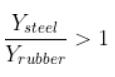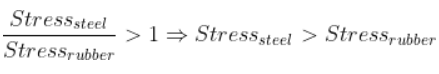9.15. Is stress a vector quantity?

Stress is a neither a scalar quantity nor a vector quantity. It is a tensor quantity. This is because stress is equal to the magnitude of the internal reaction force divided by the area of cross-section.

9.16. Identical springs of steel and copper are equally stretched. On which, more work will have to be to done?

When the identical springs of steel and copper are equally stretched, force is given as: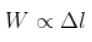Because the springs are same, their length and area will also be the same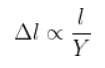From both the equations, we can say that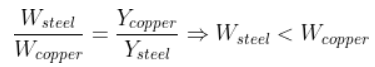9.17. What is the Young’s modulus for a perfect rigid body?

According to Hooke’s law,

Young’s modulus = Stress/ Longitudinal strain

When the given body is a perfect rigid body, then the Young’s modulus is given as: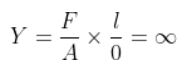9.18. What is the Bulk modulus for a perfect rigid body?

Bulk modulus is given as:

Bulk modulus = Stress/ Volume strain = pV/∆V

For a perfectly rigid body, bulk modulus is

B = pV/0 = ∞

9.19. A wire of length L and radius r is clamped rigidly at one end. When the other end of the wire is pulled by a force f, its length increases by l. Another wire of the same material of length 2L and radius 2r is pulled by a force 2f. Find the increase in length of this wire.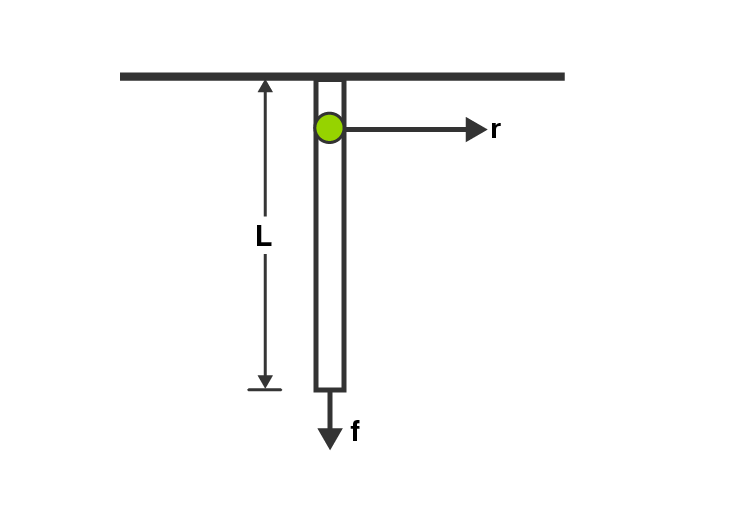From the given figure, Young’s modulus is given as

Y = (f/A)(L/l)

First case:

Length of wire = L

Force applied = f

Increase in length = l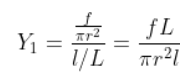Second case:

Length of wire = 2L

Force applied = 2f

Increase in length = x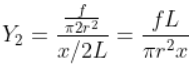Therefore, it can be said that x = l.

9.20. A steel rod of length 1 m and area of cross-section 1 cm2 is heated from 0oC to 200oC without being allowed to extend or bend. What is the tension produced in the rod?

Young’s modulus of steel, Y = 2.0 × 1011 N/m2

Coefficient of thermal expansion, α = 10-5 oC-1

Length, L = 1 m

Area of cross section A = 1 cm2 = 1×10-4 m2

Rise in temperature = 200oC

Tension produced in rod by the compression is

Y = (T/A)/(∆L/L)

T = 4 × 104 N

9.21. To what depth must a rubber ball be taken in deep sea so that its volume is decreased by 0.1%.

Bulk modulus of rubber = 9.8 × 108 N/m2

Density of sea water = 103 kg/m3

Percentage decrease in volume = 0.1%

Change in pressure, p = hρg

B = ∆P/(∆V/V)

∆P = hρg

h = 100 m

9.22. A truck is pulling a car out of a ditch by means of a steel cable that is 9.1 m long and has a radius of 5 mm. When the car just begins to move, the tension in the cable is 800 N. How much has the cable stretched?

Length of steel cable, l = 9.1 m

Tension on the cable = 800 N

Young’s modulus = Y = 2 × 1011 N/m2

Y = (F/A)/( ∆L/L)

∆L = 0.5 × 10-3 m

9.23. Two identical solid balls, one of ivory and the other of wet-clay, are dropped from the same height on the floor. Which one will rise to a greater height after striking the flood and why?

Ivory ball will rise to a greater height after striking the flood because the ivory ball is more elastic than clay ball and the amount of energy and momentum transferred is more on the ivory ball.

9.24. Consider a long steel bar under a tensile stress due to forces F acting at the edges along the length of the bar. Consider a plane making an angle θ with the length. What are the tensile and shearing stresses on this plane?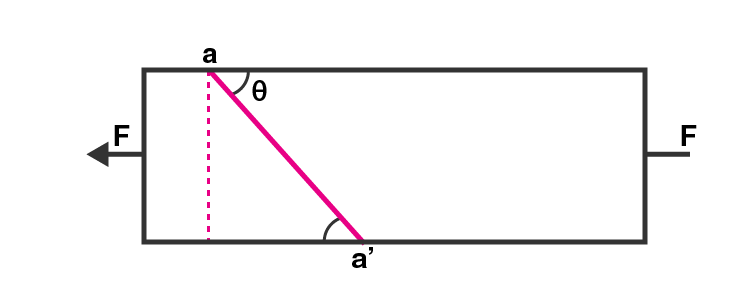a) for what angle is the tensile stress a maximum?

b) for what angle is the shearing stress a maximum?

Given,

F is applied along the horizontal

When F is perpendicular = F sin θ

When F is parallel = F cos θ

A is the cross-sectional area of the bar.

The plane aa’ is equilibrium.

Area of the plane aa’ is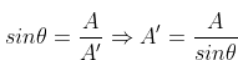Tensile stress on the plane aa’ is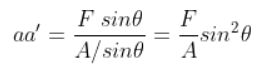Shear stress on the plane aa’ is

F sin 2θ/2A

a) When the tensile stress is maximum θ = 90o

b) When the shearing stress is maximum θ = 45o

9.25. a) A steel wire of mass μ per unit length with a circular cross-section has a radius of 0.1 cm. The wire is of length 10 m when measured lying horizontal, and hangs from a hook on the wall. A mass of 25 kg is hung from the free end of the wire. Assuming the wire to be uniform and lateral strains << longitudinal strains, find the extension in the length of the wire. The density of steel is 7860 kg/m3.

b) If the yield strength of steel is 2.5 × 108 N/m2, what is the maximum weight that can be hung at the lower end of the wire?

Let dx be the small element

Let dm be the mass

Let L be the length of the wire

Let x be the distance from the end where it is hung

Let μ be the mass per unit length

Therefore,

dm = μ.dx

r = 0.1 cm

A = π(10)-3

M = 25 kg

L = 10 m

a) The downward force is used for calculating the mass, m = 0.25 kg

b) The maximum weight that can be hung at the lower end of the wire is calculated using the tension in the wire which is the maximum at x = L

Therefore, the mass is = 78.25 kg

9.26. A steel rod of length 2l, cross-sectional area A and mass M is set rotating in a horizontal plane about an axis passing through the centre. If Y is the Young’s modulus for steel, find the extension in the length of the rod.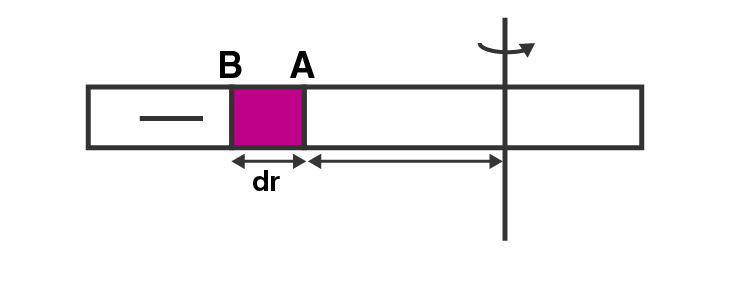T(r) and T(r+dr) are the tensions that are acting as an external force to the rod at points A and B.

T(r+dr)-T(r) is the centrifugal force on the element due to tension difference

dr = dm.rω2 is the centripetal force due to rotation on element

When the below equation is integrated,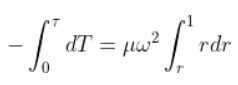We get the extension in the rod from both the sides as: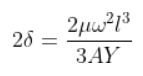9.27. An equilateral triangle ABC is formed by two Cu rods AB and BC and one Al rod. It is heated in such a way that the temperature of each rod increases by ∆T. Find the change in the angle ABC.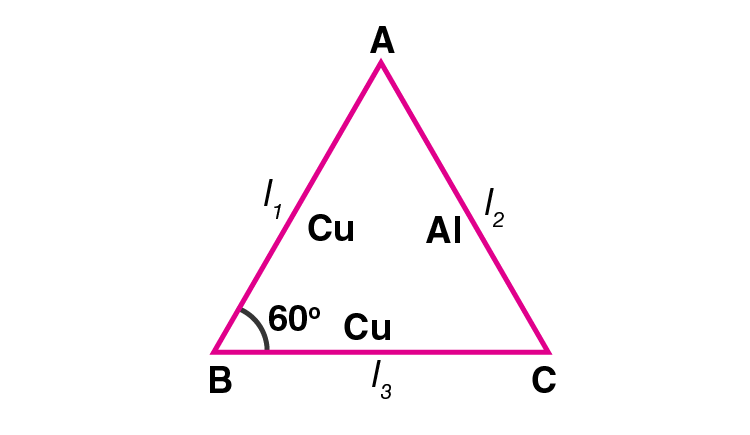Trigonometry is used for determining the change in the angle ABC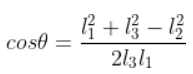When the above equation is differentiated, the change in the angle ABC is given as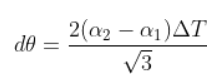9.28. In nature, the failure of structural members usually result from large torque because of twisting or bending rather than due to tensile or compressive strains. This process of structural breakdown is called buckling and in cases of tall cylindrical structures like trees, the torque is caused by its own weight bending the structure. Thus the vertical through the centre of gravity does not fall within the base. The elastic torque caused because of this bending about the central axis if the tree is given by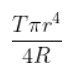. Y is the Young’s modulus, r is the radius of the trunk and R is the radius of curvature of the bent surface along the height of the tress containing the centre of gravity. Estimate the critical height of a tress for a given radius of the trunk.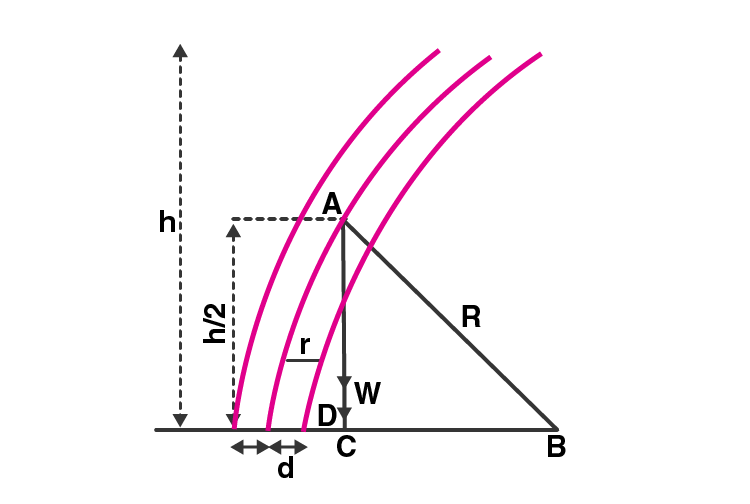Using Pythagoras theorem,

R2 = (R – d)2 + (h/2)2

Solving the equation, we get d as

d = h2/8R

W0 is the weight of the trunk per unit volume

The weight of the trunk is = (Volume)(W0)

Substituting the values for volume and weight, we can calculate the critical height, h as

h = [2Y/W0]1/3 r2/3

9.29. A stone of mass m is tied to an elastic string of negligible mass and spring constant k. The unstretched length of the string is L and has negligible mass. The other end of the string is fixed to a nail at a point P. Initially, the stone is at the same level as the point P. The stone is dropped vertically from point P.

a) find the distance y from the top when the mass comes to rest for an instant, for the first time

b) what is the maximum velocity attained by the stone in this drop?

c) what shall be the nature of the motion after the stone has reached its lowest point?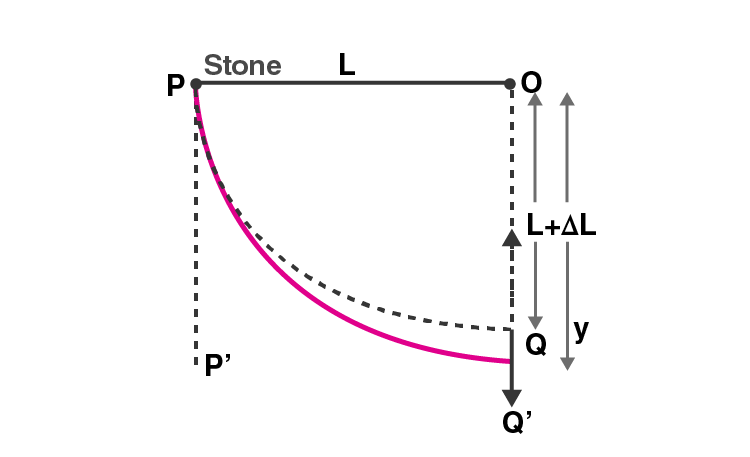PE of stone = mechanical energy of string

mgy = ½ K(y-L)2

Where K is the spring constant

L is the length of the string

Solving the above equation we get

Ky2 – 2(KL + mg)y + KL2 = 0

Using the above equation, we can determine the distance y from the top when the mass comes to rest for an instant

D = b2 – 4ac

Where a = K, b = -2(KL +mg) and c = KL2

Substituting the values we get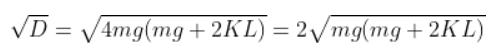Therefore, y is solved as: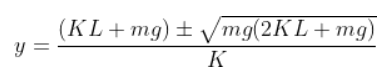b) When the velocity is maximum, acceleration is zero and therefore the force is also zero.

Let v be the maximum velocity of the stone.

The maximum velocity attained by the stone is determined with the help of the law of conservation of energy

PE lost by the stone from p to Q’ = KE of the stone + PE gained by the string

mg (L + x) = 1/2 mv2 + 1/2 Kx2

Solving the above equation gives maximum velocity v as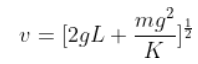c) The nature of the motion after the stone has reached its lowest point is z0

z0 = [L + mg/K]

### Why Opt for BYJU’s?

BYJU’S provide you with elegant study materials, notes, sample papers, MCQ’s, short and long answer questions, exemplar problems and worksheets. These will assist you in equipping better to face class 11 examinations and all-important engineering and medical entrance examination.